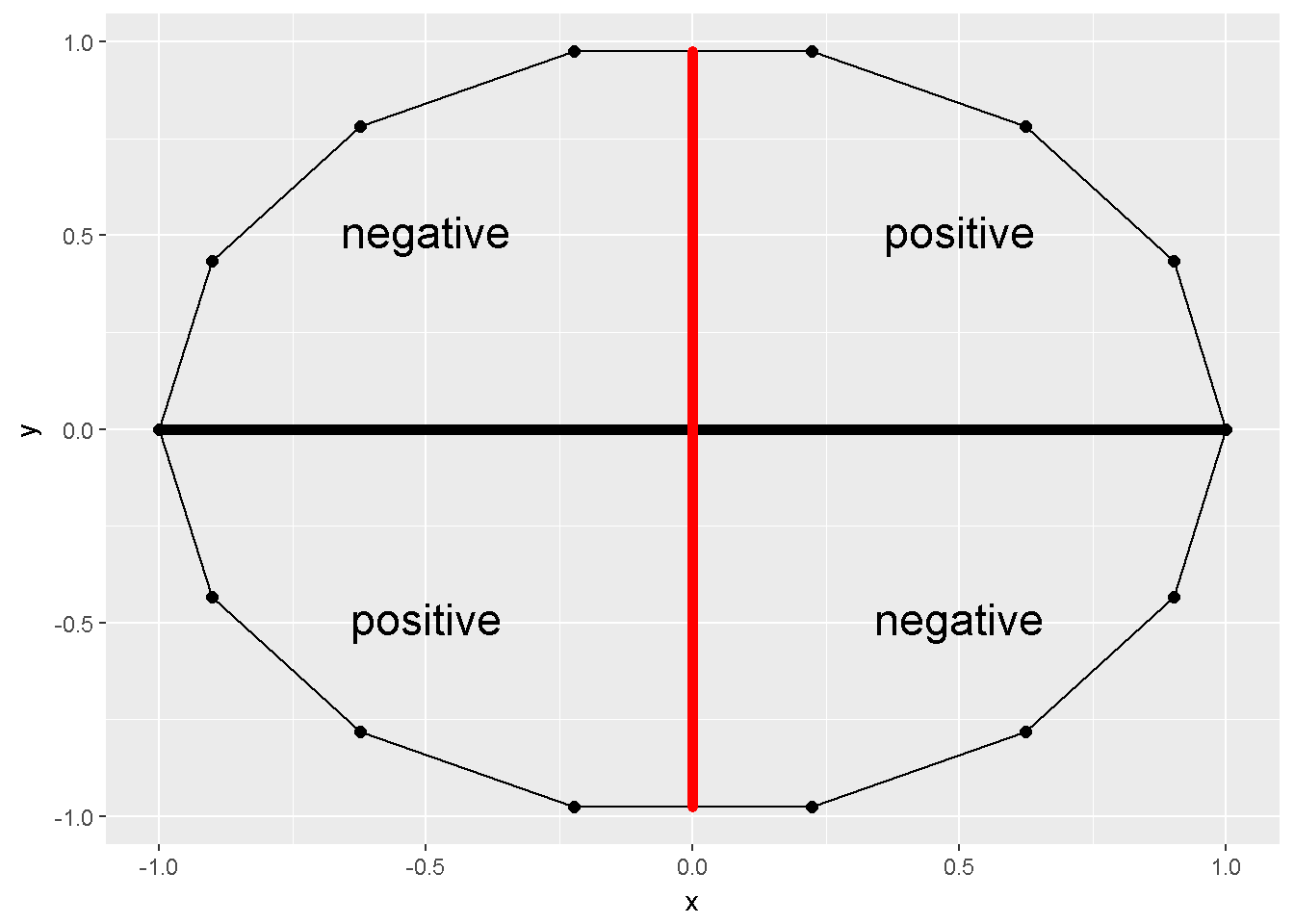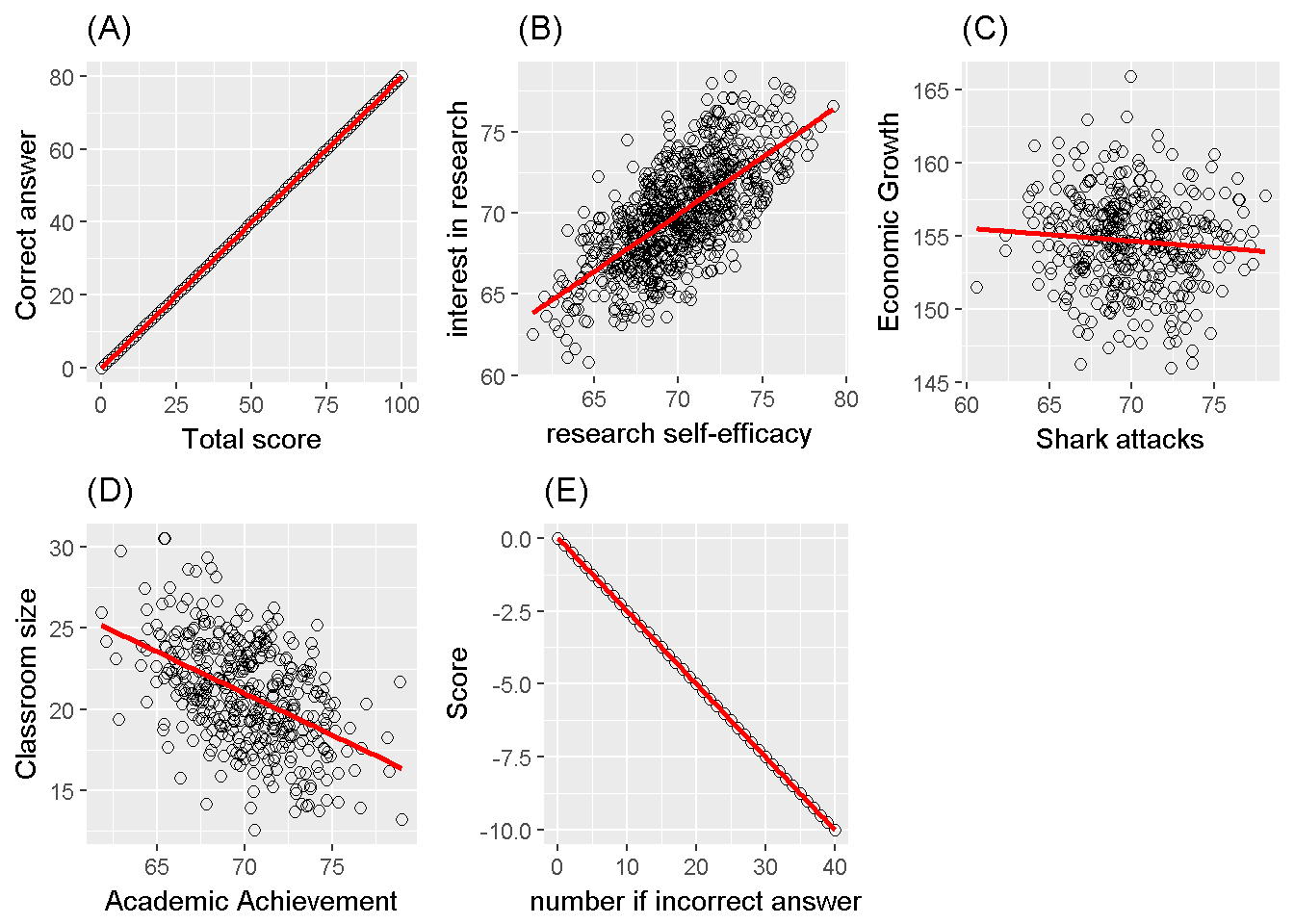# 10 Correlation

In our chapter 7, we introduced descriptive statistics; mean, variance, median, kurtosis, etc. These descriptive statistics aimed to ease the communication for a single variable. In other words, instead of transferring the entire raw data set to a colleague (or to a machine), providing these descriptives is generally satisfying and easier. However when the interest is in the association between variables, other measures are needed.

The sum of cross products, $$S_{XY}=\sum(X-\bar X)(Y- \bar Y)$$, can provide some information about the association. For example Figure 10.1 depicts an X and a Y variable. The sum of cross products for these two variables is zero.

##        x     y deviationX deviationY crossPRODUCT
## 1   1.00  0.00       0.93       0.00         0.00
## 2   0.90  0.43       0.83       0.43         0.36
## 3   0.62  0.78       0.56       0.78         0.44
## 4   0.22  0.97       0.16       0.97         0.15
## 5  -0.22  0.97      -0.29       0.97        -0.28
## 6  -0.62  0.78      -0.69       0.78        -0.54
## 7  -0.90  0.43      -0.97       0.43        -0.42
## 8  -1.00  0.00      -1.07       0.00         0.00
## 9  -0.90 -0.43      -0.97      -0.43         0.42
## 10 -0.62 -0.78      -0.69      -0.78         0.54
## 11 -0.22 -0.97      -0.29      -0.97         0.28
## 12  0.22 -0.97       0.16      -0.97        -0.15
## 13  0.62 -0.78       0.56      -0.78        -0.44
## 14  0.90 -0.43       0.83      -0.43        -0.36
## 15  1.00  0.00       0.93       0.00         0.00Figure 10.1: Sum of cross products=0

The covariance between two variable is simply $$Cov_{XY}=S_{XY}/n-1$$, but its a scale dependent measure, the correlation coefficient on the other hand generally has its bounds.

## 10.1 Pearson correlation coefficient

Pearson introduced a correlation coefficient in 1896. This coefficient ranges between -1 and +1, can be calculated as $$Cov_{XY}/S_X S_Y$$. This coefficient measures the linear relationship between two variables. Figure 10.1 depicts a correlation of zero. Even though X and Y in this figure are related to form a 14-sided polygon, the relation is not linear. Hence the correlation is zero. Figure 10.2 depicts several other associations; (A) is a perfect positive linear relationship, (B)is a positive correlation of .7, (C) substantially no linear relation, (D) is a correlation of -.4 and (E) is a correlation of -1.Figure 10.2: Correlation examples

### 10.1.1 Inference on a Pearson correlation coefficient

Information from the sample ($$r$$) can be utilized to make judgement about the population ($$\rho$$).

The z transformation , assuming a bivariate normality and a sample size of at least 10 (Myers et al. (2013)), is a helpful procedure to reach a judgement. The transformation equation is; $z_r = \frac{1}{2}ln \left( \frac{1+r}{1-r} \right)$

The standard error is; $\sigma_r = \frac{1}{\sqrt{n-3}}$

Hence the confidence intervals are $$z_r \pm z_{\alpha / 2} \sigma_r$$. Back transformation is needed to make interpretation about the correlation coefficient; $$r=\frac{e^{2z_r}-1}{e^{2z_r}+1}$$.

Utilizing a normal distribution, a null hypothesis can be tested; $z=\frac{z_r - z_{\rho_{null}}}{\frac{1}{\sqrt{n-3}}}$ The t distribution can also be utilized to test $$H_0:\rho=0$$.

$t=r\sqrt{\frac{n-2}{1-r^2}}$

The distribution for this statistic follows a t distribution with a degrees of freedom of $$n-2$$.

### 10.1.2 R codes for Pearson Correlation coefficent

For illustrative purposes we selected the city of Bayburt. The Pearson correlation is computed for the association between the Gender Attitudes scores and the annual income per person. The income per person is calculated as “total household income” divided by the “total number of residents in the house”.

# load csv from an online repository
urlfile='https://raw.githubusercontent.com/burakaydin/materyaller/gh-pages/ARPASS/dataWBT.csv'
dataWBT_Bayburt=dataWBT[dataWBT$city=="BAYBURT",] #hist(dataWBT_Bayburt$income_per_member)
## wgl
##   1